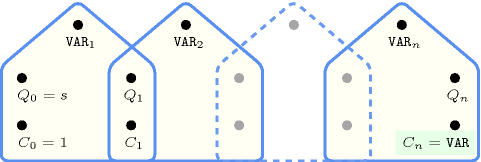## 5.30. and

Origin

Logic

Constraint

$\mathrm{𝚊𝚗𝚍}\left(\mathrm{𝚅𝙰𝚁},\mathrm{𝚅𝙰𝚁𝙸𝙰𝙱𝙻𝙴𝚂}\right)$

Synonym

$\mathrm{𝚛𝚎𝚕}$.

Arguments
 $\mathrm{𝚅𝙰𝚁}$ $\mathrm{𝚍𝚟𝚊𝚛}$ $\mathrm{𝚅𝙰𝚁𝙸𝙰𝙱𝙻𝙴𝚂}$ $\mathrm{𝚌𝚘𝚕𝚕𝚎𝚌𝚝𝚒𝚘𝚗}\left(\mathrm{𝚟𝚊𝚛}-\mathrm{𝚍𝚟𝚊𝚛}\right)$
Restrictions
 $\mathrm{𝚅𝙰𝚁}\ge 0$ $\mathrm{𝚅𝙰𝚁}\le 1$ $|\mathrm{𝚅𝙰𝚁𝙸𝙰𝙱𝙻𝙴𝚂}|\ge 2$ $\mathrm{𝚛𝚎𝚚𝚞𝚒𝚛𝚎𝚍}$$\left(\mathrm{𝚅𝙰𝚁𝙸𝙰𝙱𝙻𝙴𝚂},\mathrm{𝚟𝚊𝚛}\right)$ $\mathrm{𝚅𝙰𝚁𝙸𝙰𝙱𝙻𝙴𝚂}.\mathrm{𝚟𝚊𝚛}\ge 0$ $\mathrm{𝚅𝙰𝚁𝙸𝙰𝙱𝙻𝙴𝚂}.\mathrm{𝚟𝚊𝚛}\le 1$
Purpose

Let $\mathrm{𝚅𝙰𝚁𝙸𝙰𝙱𝙻𝙴𝚂}$ be a collection of 0-1 variables ${\mathrm{𝚅𝙰𝚁}}_{1},{\mathrm{𝚅𝙰𝚁}}_{2},\cdots ,{\mathrm{𝚅𝙰𝚁}}_{n}$ $\left(n\ge 2\right)$. Enforce $\mathrm{𝚅𝙰𝚁}={\mathrm{𝚅𝙰𝚁}}_{1}\wedge {\mathrm{𝚅𝙰𝚁}}_{2}\wedge \cdots \wedge {\mathrm{𝚅𝙰𝚁}}_{n}$.

Example
 $\left(0,〈0,0〉\right)$ $\left(0,〈0,1〉\right)$ $\left(0,〈1,0〉\right)$ $\left(1,〈1,1〉\right)$ $\left(0,〈1,0,1〉\right)$
Symmetry

Items of $\mathrm{𝚅𝙰𝚁𝙸𝙰𝙱𝙻𝙴𝚂}$ are permutable.

Arg. properties
• Functional dependency: $\mathrm{𝚅𝙰𝚁}$ determined by $\mathrm{𝚅𝙰𝚁𝙸𝙰𝙱𝙻𝙴𝚂}$.

• Extensible wrt. $\mathrm{𝚅𝙰𝚁𝙸𝙰𝙱𝙻𝙴𝚂}$ when $\mathrm{𝚅𝙰𝚁}=0$.

• Aggregate: $\mathrm{𝚅𝙰𝚁}\left(\wedge \right)$, $\mathrm{𝚅𝙰𝚁𝙸𝙰𝙱𝙻𝙴𝚂}\left(\mathrm{𝚞𝚗𝚒𝚘𝚗}\right)$.

Counting
 Length ($n$) 2 3 4 5 6 7 8 Solutions 4 8 16 32 64 128 256

Number of solutions for $\mathrm{𝚊𝚗𝚍}$: domains $0..n$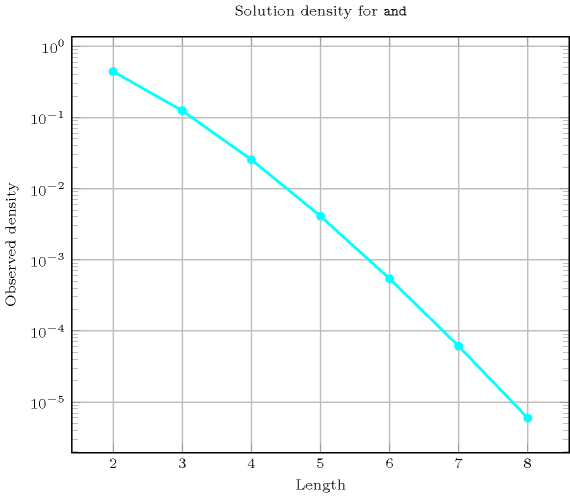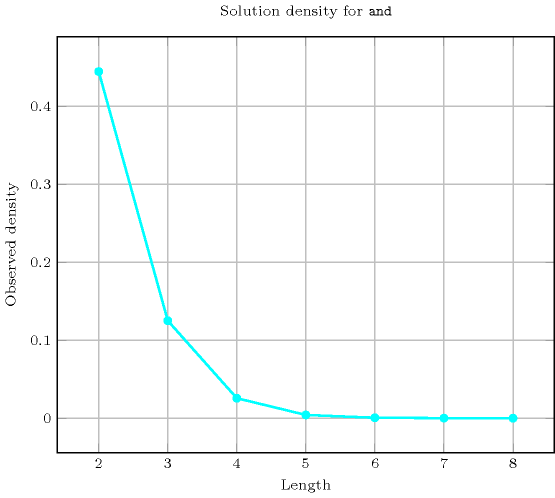Length ($n$)2345678
Total48163264128256
 Parameter value

037153163127255
11111111

Solution count for $\mathrm{𝚊𝚗𝚍}$: domains $0..n$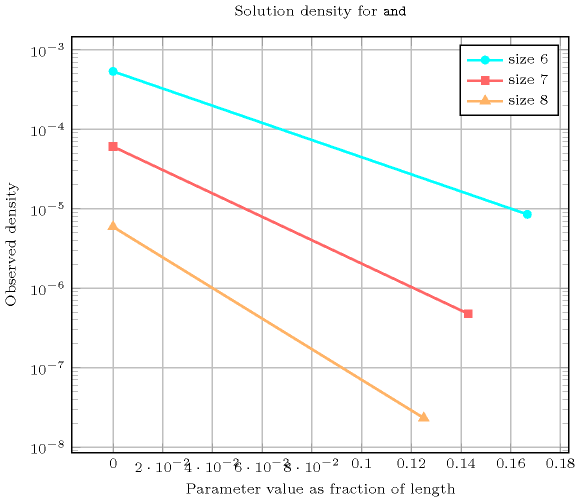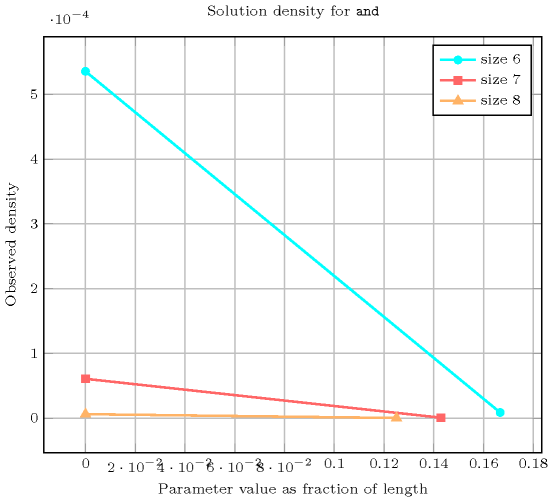Systems

reifiedAnd in Choco, rel in Gecode, andbool in JaCoP, #/\ in SICStus.

Keywords
Cond. implications

$•$ $\mathrm{𝚊𝚗𝚍}\left(\mathrm{𝚅𝙰𝚁},\mathrm{𝚅𝙰𝚁𝙸𝙰𝙱𝙻𝙴𝚂}\right)$

with  $|\mathrm{𝚅𝙰𝚁𝙸𝙰𝙱𝙻𝙴𝚂}|>2$

implies $\mathrm{𝚜𝚘𝚖𝚎}_\mathrm{𝚎𝚚𝚞𝚊𝚕}$$\left(\mathrm{𝚅𝙰𝚁𝙸𝙰𝙱𝙻𝙴𝚂}\right)$.

$•$ $\mathrm{𝚊𝚗𝚍}\left(\mathrm{𝚅𝙰𝚁},\mathrm{𝚅𝙰𝚁𝙸𝙰𝙱𝙻𝙴𝚂}\right)$

with  $\mathrm{𝚅𝙰𝚁}=0$

implies $\mathrm{𝚗𝚊𝚗𝚍}$$\left(\mathrm{𝚅𝙰𝚁},\mathrm{𝚅𝙰𝚁𝙸𝙰𝙱𝙻𝙴𝚂}\right)$

when  $\mathrm{𝚅𝙰𝚁}=1$.

$•$ $\mathrm{𝚊𝚗𝚍}\left(\mathrm{𝚅𝙰𝚁},\mathrm{𝚅𝙰𝚁𝙸𝙰𝙱𝙻𝙴𝚂}\right)$

with  $\mathrm{𝚅𝙰𝚁}=1$

implies $\mathrm{𝚗𝚊𝚗𝚍}$$\left(\mathrm{𝚅𝙰𝚁},\mathrm{𝚅𝙰𝚁𝙸𝙰𝙱𝙻𝙴𝚂}\right)$

when  $\mathrm{𝚅𝙰𝚁}=0$.

Automaton

Figure 5.30.1 depicts a first deterministic automaton without counter associated with the $\mathrm{𝚊𝚗𝚍}$ constraint. To the first argument $\mathrm{𝚅𝙰𝚁}$ of the $\mathrm{𝚊𝚗𝚍}$ constraint corresponds the first signature variable. To each variable ${\mathrm{𝚅𝙰𝚁}}_{i}$ of the second argument $\mathrm{𝚅𝙰𝚁𝙸𝙰𝙱𝙻𝙴𝚂}$ of the $\mathrm{𝚊𝚗𝚍}$ constraint corresponds the next signature variable. There is no signature constraint.

##### Figure 5.30.1. Counter free automaton of the $\mathrm{𝚊𝚗𝚍}$$\left(\mathrm{𝚅𝙰𝚁},〈{\mathrm{𝚅𝙰𝚁}}_{1},{\mathrm{𝚅𝙰𝚁}}_{2},\cdots ,{\mathrm{𝚅𝙰𝚁}}_{n}〉\right)$ constraint (the transition $i\stackrel{{\mathrm{𝚅𝙰𝚁}}_{i}=0}{\to }k$ represents the fact that at least one variable ${\mathrm{𝚅𝙰𝚁}}_{i}$ should be set to 0 when $\mathrm{𝚅𝙰𝚁}=0$, while the transition $j\stackrel{{\mathrm{𝚅𝙰𝚁}}_{i}=1}{\to }j$ represents the fact that all ${\mathrm{𝚅𝙰𝚁}}_{i}$ should be set to 1 when $\mathrm{𝚅𝙰𝚁}=1$)##### Figure 5.30.2. Hypergraph of the reformulation corresponding to the automaton of the $\mathrm{𝚊𝚗𝚍}$ constraintFigure 5.30.3 depicts a second deterministic automaton with one counter associated with the $\mathrm{𝚊𝚗𝚍}$ constraint, where the argument $\mathrm{𝚅𝙰𝚁}$ is unified to the final value of the counter.

##### Figure 5.30.3. Automaton (with one counter) of the $\mathrm{𝚊𝚗𝚍}$ constraint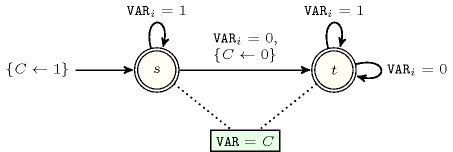##### Figure 5.30.4. Hypergraph of the reformulation corresponding to the automaton (with one counter) of the $\mathrm{𝚊𝚗𝚍}$ constraint (since all states of the automaton are accepting there is no restriction on the last variable ${Q}_{n}$)